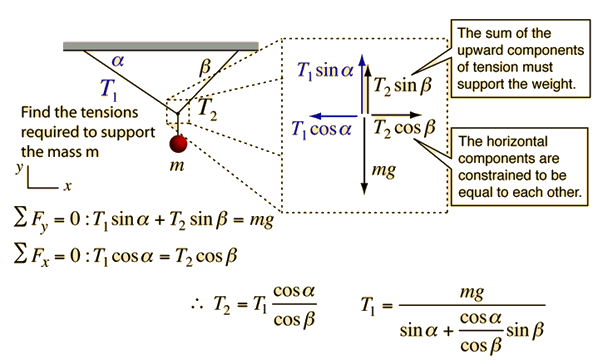# Force Equilibrium Example

Force equilibrium problems like this can be analyzed by drawing a free-body diagram of the point of attachment of the mass m, since it must be at equilibrium. The tensions should be resolved into horizontal and vertical components to apply the force equilibrium condition.An object of mass kg and weight N can be supported by the following combination of tensions and angles of cables:

 Tension T1 =N, α = degrees Tension T2 = N, β =degrees
You may explore the effect of changing the angles upon the required tensions in the cables. Making both angles smaller will increase the required tensions. Why is that?
Index

Force equilibrium examples

 HyperPhysics***** Mechanics R Nave
Go Back